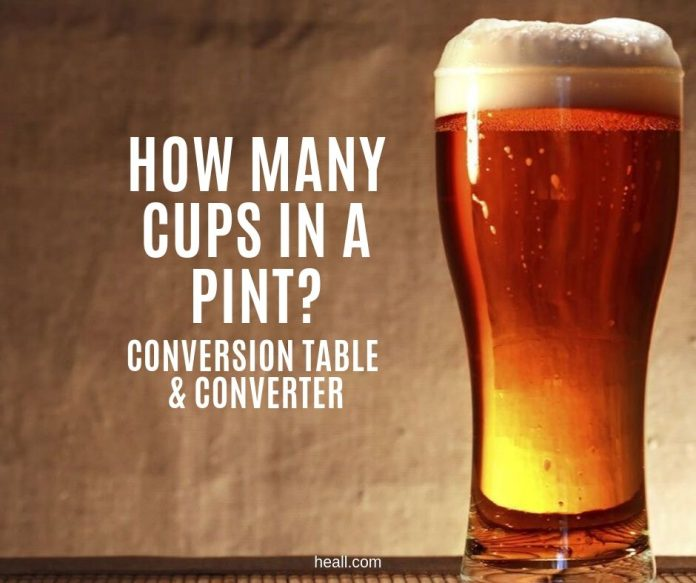# How many cups in a pint?

0
2783Mixing and matching units of measurement can be a real challenge if you do not know that, one, they differ in conversion values depending on where you are in the world and two, there are dry and liquid conversions.

A cup in a pint and vice-versa is not an exception. So fundamentally, we must know the conversion value of cups in a pint and according to the standard conversion, there are 2 cups in a pint. Now let’s build on this information.

1 pint = 2 cups

[CP_CALCULATED_FIELDS id=”28″]

## What is a Pint?

Before we could know how many ounces are in a pint, we must understand what a pint is first. Pint is used in measuring volume or fluid capacity. It is expressed using the abbreviation p and it is used in both UK/imperial and US metric system.

A pint is equal to one-half quart or one-eighth gallon. It is usually used for liquids such as milk, water, wine and beer.

## Is a cup bigger than a pint?

Both cups and pint measures volume capacity and it may be essential to know which of them is bigger than the other. According to the Monterey Institute, the pint is bigger than the cup.

To prove that, let us follow this explanation. One pint is equal to two cups but one cup is only equal to 0.5 pint. If there are two cups in a pint and less than one pint in a cup, indeed, the pint is bigger than a cup.

## How many cups in a pint?

Knowing how many cups in a pint is very essential for cooking, specifically in measuring liquid ingredients. As has been mentioned, cups in a pint depend on factors such as the metric system (if it is US or imperial/UK) and whether it is dry or liquid measurement. Take this for example:

 1 US liquid pint 2 US cups 1 US liquid pint 1.66 UK/imperial cups 1 UK/imperial pint 2 UK/imperial cups 1 UK/imperial pint 2.37 US cups

## How many cups in a pint of alcohol?

Now, this is a tricky question for two reasons. First, alcohol is expressed in fluid ounces, not cups. So we first need to convert its volume capacity to pints first before we could get with the cup value. Second, what alcoholic beverage are we talking about? To illustrate this, refer to the sample table below.

 Amount of Alcohol in standard drinks Fluid ounce Pint Cup Beer or wine coolers 5 0.31 0.61 Malt 7 0.43 0.85 Cider 6 0.37 0.73 Wine 12 0.75 1.48

According to Very Well Mind, standard drinks have different alcohol contents. Since we want to know how many cups in a pint of alcohol, we need to understand the context in which this question can be variably answered.

## How many cups in a pint of blueberries?

According to My Recipes, there are 16 ounces in a pint of blueberries. If we follow this conversion, it would mean that there is one cup in a pint of blueberries.

However, it also says that this is quite misleading because there are really just 12 ounces in a pint. Thus, if there are only really 12 ounces in a pint, then in actuality, a pint of blueberries will just weigh 0.75 cups.

1 pint of blueberries = 0.75 cups

## Conclusion

In conclusion, cup to pint is interesting because sometimes, there are no available direct conversions for it. But the good thing about this conversion is that it can be used for a lot of everyday things, from dry to liquid ingredients to social life like in drinking.